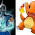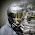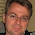## Monday, March 18, 2013

### Historgrams and Overlayed Normal Curves in Excel

How to create histograms using Excel 2003 and 2007.

We need a variable column (all in numeric value), the example has values from cell A2 to A101.

Now we can calculate different this by simply developing formula (some of them are not must !)

Formula to calculate maximum [ value in C2 cell above]
=MAX(A2:A101)

Formula to calculate minimum [value in C3 cell above ]
=MIN(A2:A101)

The range can be calculated by subtracting Minimum from Maximum value [ value in C4 cell above]

If you want to create 10 bins, you can calculate bin interval by: [ value in C5 cell above]

= range /10

Now we can fill the bin column, where the first number is minimum and is increased by the bin interval specified above.

=(D2+\$C\$5)

Here C5 cell has bin interval. Now by just pasting formula down we can fill the bin interval.

Bin interval can also be just users own choice.

Plotting histogram:

(1) In Excel 2003:

We need to generate frequency table for bins stored. Let's just copy and paste the bins calculated in separate column (use paste special and paste values only). New column called frequency:

(1) Highlight the range of cells that are supposed to hold the frequency counts (L2:L11). These will be every Frequency Count cells next to the bin increments.

(2) Choose Insert, then Function, pick the Statistical Function category and scroll down in the box and choose FREQUENCY as the Function name. Now pick the Data_array and Bins_array range. See the range of the trait in A2:A101  Data_array column while Bins arrays is in K2:K11 cells. The click OK.

We are not done yet, we need to calculate the frequency down, in the frequency count cells. Please note that  applying function (copying cell) here is a bit different. With Frequency cell highlighted K2:K11, click the  on the FREQUENCY function into the formula bar ( =FREQUENCY(A2:A101,K2:K11)) and the apply the function by typing  Control-Shift-Enter on a PC (type Command-Return on the Mac).

Now it will fill the frequency for each bin. Now we can use this to plot histogram. We can use bar plot, however, the the bin names should be converted as text like features, use and apply the following type of formula to all frequency cells by generating new cell freq1 column.

=(ROUND(K2, 1)&"")

Then we bin1 column and freq column to generate column (bar) plot as usual. You can change spacing between bars to make the bars look compact.

However in 2007 Excel provides more automated function to generate frequency table and histograms.

(B) In Excel 2007
The function histogram can be used to generate Bin and Empirical Frequency and generates a bar chart (histogram).

Click in the data analysis menu, click histogram. Enter input data range and Bin Range.
You can provide output range or select new worksheet or workbook. Click chart output to plot the histogram.

Now the Raw histogram is available, you can polish this in the way you like ! For example removing the gaps to make it compact.

Plotting the theoretical distribution (normal distribution curve):

To plot the theoretical normal distribution curve we need to specify mean and standard deviations. Unless we have known or assumed mean and standard deviation, we can simply calculated this from the sample we have,  let's first determine mean, standard deviation. I do not want to go on theoretical side of what we should use as mean or standard deviation.

Formula for:
Mean for the trait 1
=AVERAGE(A2:A101)
When we have assumed or known population mean we can replace this value with the assumed or known population mean.

Standard deviation for trait 1
=STDEV(A2:A101)
When we have assumed or known population standard deviation we can replace this value with the assumed or known population standard deviation.

Formula to calculate maximum
=MAX(A2:A101)

When we have assumed or known population mean and standard deviation we can replace this value with a defined value: for example value of mean + 3*standard deviation gives us 99% area under curve. We can set slightly higher maximum than this may be mean + 3.1*standard deviation.

Formula to calculate minimum
=MIN(A2:A101)

When we have assumed or known population mean and standard deviation we can replace this value with a defined value: for example value of mean - 3*standard deviation gives us 99% area under curve. We can set slightly higher maximum than this may be mean - 3.1*standard deviation.

Number of samples used to calculate above mean and standard deviation (counting non-empty cells):
=COUNT(A2:A101)

interval bin cell here is for the normal curve. The higher numbers is recommended to produce smooth normal curve (more close to theoretical). Here we want to plot the curve between mean + or - 3 x Standard deviation and we want to supply 200 bins to create the smooth curve. Thus the bin becomes:

= ((F2+3*F1) - (F2-3*F1)) / 200

User can choose own bin value (usually should be small and relative to mean and sd of the data).

For value of X,

=(G1)

Then the following values will be increased by the bin interval calculated in G3 cell.

=(H2+\$G\$3)

Now we need to fill the theoretical frequency:

The formula for the first cell stand like the following, paste the formula down:

=NORMDIST(H2, \$F\$2,\$F\$1, FALSE )*\$F\$4

Here \$F\$4 stands for the total number of samples.  \$F\$2 is mean and \$F\$1 standard deviation. If we want to plot distribution that is standardized (mean = 0, sd =1), then we do not need this term. Multiplying is important when overlaying over total number type histogram.

Now you can simply plot X, Y smoothed line plot:

We can modify this curve to fit the our needs and taste.

Overlay the normal curve over the histogram:

(a) Excel 2003.
Plot the normal distribution as discussed above. Now we need a trick to add the bars. Regular bar plot do not support continuous  x and y values, so we need to use error bar. The only limitation here is bar size can not be increased to very large (in contrast to excel 2007).

(1) First draw the normal distribution curve

(2) Add new data to add the empirical bin interval and their frequency (to be used for bar plot). Right click on the plot to add the data to the plot. Under series, click add a new series. Then select X range where the bin (not bin1) is stored and frequency in Y value.

(3) Click the new line added and right click and format the series to add error bars to the plot. Put 100 under percentage. Click minus side of error ba.

Click OK and now we have got a way plot. Remove the curve of line by selecting line type non under  format data series menu, that is not needed any more. We can click the bars and increase bar size to some extent.

(B) Using Excel 2007
The advantages of using excel 2007 is that we can change bar width to look more like histogram.
(1) Generate histogram as discussed above (we do not need a plot rather we need frequency distribution).

(2) Create the theortical curve as discussed above.

(2) In addition to the regular curve add a new curve using the bin and frequency.  By adding data series to above plot.  Select data and then add the series.

(3) Now click the recently added series (which is emperical density curve) and add error bars from the layout menu and then error bars and then select more error bar options. Then change the setting as of the following figure.

(4) Now you can delete the horizontal bars and increase the size of the bar, to make it look like histogram. Also you can supress the line by selecting no line opition.

#### 17 comments:

1.Would you please explain how do you get the values in G1, G2, G3?

I am really confused.

1.see the recent edits if this helps.

2.I agree with Jabzero ManN. This is confusing. How do you select the value of G1 to be -1.44034? What about value G2?

3.Answer:
Sign correction on above
G1=(F2-3*F1)
G2=(F2+3*F1)
G3= ((F2+3*F1) - (F2-3*F1)) / 200

2.In the "Plotting the theoretical distribution (normal distribution curve)"

3.this was AWESOME! thanks!

4.How do you get G1 :S

5.I am confused as to where the Normal column and the numbers for the X column came from. How was it obtained?

6.Hi, my norm dist curve end up having a height of 2500 on the mean point, but my histogram plot is only 180... what could i have done wrong ?

7.graph yang menarik
ini mudah dipahami
terima kasih infonya

8.Is the example data available?

9.This really helped, Thanks

10.Hi, for this formula : =NORMDIST(H2, \$F\$2,\$F\$1, FALSE )*\$F\$4
Why do we need to multiply by 100?
What happen if the histogram and bell curve has different max point? the chart look weird. is this ok?

11.I found one more Blog about Microsoft Excel which is very helpful in learning Advance Excel.

12.material handling equipments | Automated Storage & Retrieval System | Conveyors | Automated Guided Vehicles | Warehouse Management System | SCADA Supervision Software

13.i am from USA, i am here to give my testimony how i was cured from HIV, i
contacted my HIV via blade. a friend of my use blade to peel of her
finger nails and drop it where she use it, so after she has left i did know
what came unto me i looked at my nails, my nails were very long and i took
the blade which she just used on her own nails to cut of my finger nails,
as i was maintaining my names, i mistakenly injured myself. i did even
bothered about it, so when i got to the hospital the next week when i was
ill the doctor told me that i am HIV positive, i wondered where did i got
it from so i remembered how i use my friend blade to cut off my hand so i
feel so sad in my heart to the extent that i don’t even know what to do, so
one day i was passing through the internet i met a testimony of a lady that all
talk about how she was cured by a doctor called DR Imoloa so i quickly
emailed the doctor email, and he also replied to me an told me the
requirements which i will provide and I do according to his command,
he prepare a herbal medicine for me which i took. he
called me the next week that i should go for a test which i did to my own
surprise i found that i was HIV negative. Thanks to him once more the great
doctor that cured me DR Imoloa so you can also emailed him at
{drimoloaherbalmademedicine@gmail.com} or whatssapp him on +2347081986098} God Bless you Sir.

14.Dr. Imoloa has really made me so much believe in him by getting me cured with his herbal treatment. i really appreciate you Dr.imoloa for bringing back happiness to my life again. thank you so much,friends join me to thank him for what he has actually done for me i pray to you all for a good life and good health, and most especially to you Dr. imoloa Thanks

I have been suffering from (HERPES SIMPLEX VIRUS) disease for the past four years and had constant pain, especially in my knees. During the first year,I had faith in God that i would be healed someday.This disease started circulating all over my body and i have been taking treatment from my doctors, few months ago i came on search on the internet if i could get any information concerning the cure of this disease, on my search i saw a testimony of someone who has been healed from (HERPES SIMPLEX VIRUS) by this Man Dr imoloa and she drop the email address of this man and advise we should contact him for any sickness that he would be of help, so i wrote to Dr. imoloa telling him about my (HERPES Virus) well after all the procedures and remedy given to me by this man few weeks later i started experiencing changes all over me. I am now here to testify that i am no longer a herpes patient, I have experience a total transformation in my life,for all herpes patients get your herbal medicine to cure your sickness. And there has being rapid improvement in my health, I no longer feel pains and I wake up each morning feeling revived. So friends my advise is if you have such sickness or any other at all,you can contact him on drimolaherbalmademedicine@gmail.com, you can still reach him on whatssap- +2347081986098
CANCER
EPILEPSY.
GENPILENCIN.
HIV AIDS.
DIABETICS
STROKE.
EXPANDS OF PENIS BREAST
H.P.V TYPE 1 TYPE 2 TYPE 3 AND TYPE 4. TYPE 5.
HUMAN PAPAILOMA VIRUS.
HERPES.
SYPHILIS.
HEPATITIS A B and C.